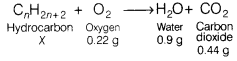# Calculate the mass of hydrocarbon used

Complete combustion of a hydrocarbon in 0.22 g oxygen produces 0.9 g of water and 0.44 g of CO2. Calculate the mass of hydrocarbon used.

The chemical equation for the above reaction is as follows:From law of conservation of mass, we have
x + 0.22 = 0.9 + 0.44
x = 1.34 - 0.22 = 1.12 g